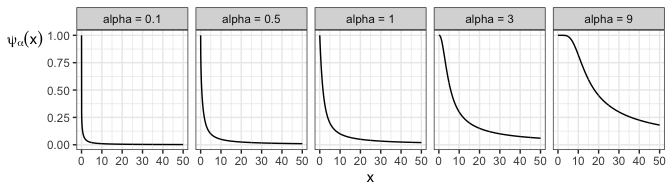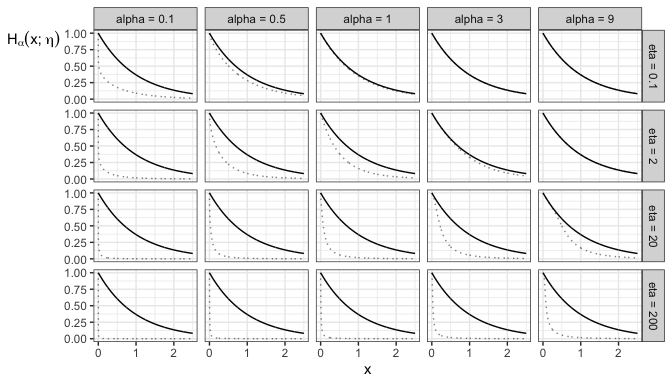# Formulas for the IG and IGL Copula Families

The IG and IGL copula families (short for “Integrated Gamma” and “Integrated Gamma Limit”) copula families are special cases of a wider class of copulas, and most of the formulas for IG and IGL copula quantities don’t simplify from their more general form. - The IGL copula family is a special case of a class of copulas first defined by Durante and Jaworski (2012) (hence is referred to as the DJ copula class). - The IG copula family is a special case of an interpolated version of the DJ copula class, first described by Coia (2017).

We’ll start with formulas for a general DJ copula and its interpolated copulas, and end with formulas specific to the IG and IGL copula families, where relevant.

# 1 General Definition

## 1.1 Definition via cdf

Both the DJ and the Interpolated DJ copula classes (and thus the IG and IGL copulas) are characterized by a generating function $$\psi:[0, \infty) \rightarrow (0, 1]$$, which is a concave distribution function or convex survival function.

A DJ copula (and thus an IGL copula family) has cdf $C_{\text{DJ}}(u, v; \psi) = u + v - 1 + (1 - u) \psi\left((1 - u) \psi^{\leftarrow}(1 - v)\right),$ for $$(u, v)$$ in the unit square, where $$\psi^{\leftarrow}$$ is the left-inverse of $$\psi$$.

But, this class does not contain the independence copula, so we can introduce an interpolating parameter $$\theta \geq 0$$ such that $$\theta = 0$$ results in the independence copula, and $$\theta = \infty$$ results in the DJ copula class – hence interpolating DJ copulas with the independence copula.

An interpolated DJ copula (and thus an IG copula) has cdf $C_{\text{intDJ}}(u, v; \theta, \psi) = u + v - 1 + (1 - u) H_{\psi}\left(H_{\psi}^{\leftarrow}(1 - v; \theta); (1 - u) \theta \right)$ for $$(u, v)$$ in the unit square, where $$H_{\psi}$$ is the interpolating function of $$\psi$$, defined as $H_{\psi}(x; \eta) = e ^ {-x} \psi(\eta x)$ for $$x > 0$$ and $$\eta \geq 0$$, and has a range between 0 and 1. Its derivative (with respect to the first argument) is also useful: $\text{D}_1 H_{\psi}(x; \eta) = - e ^ {-x} (\psi(\eta x) - \eta \psi'(\eta x)).$

Although the DJ copula class can be considered part of the Interpolated DJ class if we include $$\theta = \infty$$ in the parameter space, the formulas when $$\theta = \infty$$ do not simplify in an obvious way, so it’s best to treat the two classes separately.

All formulas in this vignette can be found in Coia (2017), but under a different (and more complex) parameterization: the $$\psi$$ function is $$\psi(1/x)$$ and the $$H$$ function is $$H_{\psi}(x; \theta) = \frac{1}{x} \psi(1 / (\theta \log x))$$, and the copula formulas are correspondingly slightly different.

## 1.2 Kappa Transform of $$\psi$$

Besides $$H_{\psi}$$, another transformation of $$\psi$$ is necessary for writing formulas succinctly: $\kappa_{\psi}(x) = \frac{\text{d}}{\text{d}x} x \psi(x) = \psi(x) + x \psi'(x)$ for $$x > 0$$.

By the way, not all choices of $$\psi$$ result in valid DJ / Interpolated DJ copulas – the requirement has to do with the $$\kappa$$ function. So, in case you want to go in the opposite direction, and obtain $$\psi$$ from a choice of $$\kappa$$, we can do so by solving the differential equation in the definition of $$\kappa$$, to get $\psi(x) = \frac{1}{x} \int_{0}^{x} \kappa(t) \text{d}t.$

## 1.3 Copula Quantities: DJ copula class (IGL copula family)

To simplify formulas, denote $$y = \psi^{\leftarrow}(1 - v)$$. Throughout, $$p$$, $$u$$, and $$v$$ are numbers between 0 and 1.

The density of a DJ copula (and thus an IGL copula) is $c_{\text{DJ}}(u, v; \psi) = (1 - u) \frac{\kappa_{\psi}'((1 - u) y)}{\psi'(y)}$

The two families of conditional distributions, obtained by conditioning on either the 1st or 2nd variable, are $\begin{equation} \begin{split} C_{\text{DJ}, 2 | 1}(v | u; \psi) & = 1 - \kappa_{\psi}((1 - u) y); \\ C_{\text{DJ}, 1 | 2}(u | v; \psi) & = 1 - (1 - u) ^ 2 \frac{\psi'((1 - u) y)}{\psi'(y)}. \end{split} \end{equation}$ The corresponding quantile functions are the (left) inverses of these functions, for which the 2|1 quantile function has a closed form: $C_{\text{DJ}, 2 | 1}^{-1}(p | u; \psi) = 1 - \psi\left((1 - u) ^ {-1} \kappa_{\psi}^{\leftarrow}(1 - p) \right)$

To check these equations, note that $$\frac{\text{d}y}{\text{d}v} = - 1 / \psi'(y)$$. If comparing to the formulas from Coia (2017) Section E.1.2, note that the formulas there are defined for the copula reflections.

## 1.4 Copula Quantities: Interpolated DJ copula class (IG copula family)

To simplify formulas, denote $$y = H_{\psi} ^ {\leftarrow}(1 - v; \theta)$$. Throughout, $$p$$, $$u$$, and $$v$$ are numbers between 0 and 1.

The density of an interpolated DJ copula (and thus an IG copula) is $\begin{equation} c_{\text{intDJ}}(u, v; \theta, \psi) = \frac{\text{D}_1 H_{\kappa_{\psi}}(y; (1 - u) \theta)} {\text{D}_1 H_{\psi}(y; \theta)} \end{equation}$

The two families of conditional distributions, obtained by conditioning on either the 1st or 2nd variable, have a convenient form if we consider the interpolating function $$H$$ of the $$\kappa$$ function instead of the $$\psi$$ function: $\begin{equation} \begin{split} C_{\text{intDJ}, 2 | 1}(v | u; \theta, \psi) & = 1 - H_{\kappa_{\psi}}\left(y; (1 - u) \theta \right) \\ C_{\text{intDJ}, 1 | 2}(u | v; \theta, \psi) & = 1 - (1 - u) \frac{\text{D}_1 H_{\psi}(y; (1 - u) \theta)} {\text{D}_1 H_{\psi}(y; \theta)}\\ \end{split} \end{equation}$ The corresponding quantile functions are the (left) inverses of these functions, for which the 2|1 quantile function has a closed form: $\begin{equation} \begin{split} C_{\text{intDJ}, 2 | 1}^{-1}(p | u; \theta, \psi) & = 1 - H_{\psi} \left( H_{\kappa_{\psi}} ^ {\leftarrow} (1 - p; (1 - u) \theta); \theta \right) \end{split} \end{equation}$

To check these equations, note that $$\frac{\text{d}y}{\text{d}v} = - 1 / \text{D}_1 H_\psi(y; \theta)$$.

# 2 Formulas specific to IG and IGL Copulas

## 2.1 Generating Functions

The generating functions of the IG and IGL copula families rely on the Gamma($$\alpha$$) distribution, which has cdf $F_{\alpha}(x) = \frac{\Gamma(\alpha) - \Gamma^{*}(\alpha, x)}{\Gamma(\alpha)}$ for $$\alpha > 0$$ and $$x \ge 0$$, where $$\Gamma$$ is the Gamma function, and $$\Gamma^{*}$$ is the (upper) incomplete Gamma function defined as $\Gamma^{*}(\alpha, x) = \int_x^{\infty} t ^ {\alpha - 1} e ^ {-t} \text{d} t.$ The density function is denoted by $$f_{\alpha}$$.

To emphasize the dependence of $$\psi$$, $$\kappa$$, and $$H$$ on the parameter $$\alpha$$, this parameter is written as a subscript. The $$\psi$$ function and its derivative are $\begin{equation} \begin{split} \psi_{\alpha}(x) & = 1 - F_{\alpha}(x) + \frac{\alpha}{x} F_{\alpha + 1}(x); \\ \psi_{\alpha}'(x) & = - \frac{\alpha}{x ^ 2} F_{\alpha + 1}(x). \end{split} \end{equation}$ Here are some plots of these functions for various values of $$\alpha$$.The $$\kappa$$ function is the Gamma survival function, $\kappa_{\alpha}(x) = 1 - F_{\alpha}(x).$

The $$H$$ function does not simplify, although for convenience, $$H_{\psi_{\alpha}}$$ is simply denoted $$H_{\alpha}$$. Here are some plots of these functions, compared with the plot of the negative exponential $$e^{-x}$$ as the faded dotted line. Notice that increasing $$\alpha$$ draws $$H_{\alpha}(\cdot, \eta)$$ closer to the negative exponential, whereas increasing $$\eta$$ pulls it further.

## Warning: guides(<scale> = FALSE) is deprecated. Please use guides(<scale> =
## "none") instead.## 2.2 Copula Quantities

Most of the formulas for copula quantities do not simplify nicely from their general forms. But, here are the ones that do.

For the IGL copula family: $\begin{equation} \begin{split} C_{\text{IGL}, 1 | 2}(u | v; \theta, \alpha) & = 1 - \frac{F_{\alpha + 1}((1 - u) y)}{F_{\alpha + 1}(y)}; \\ C_{\text{IGL}, 1 | 2}^{-1}(p | v; \theta, \alpha) & = 1 - \frac{1}{y} F_{\alpha + 1}^{-1}\left((1 - p) F_{\alpha + 1}(y)\right). \end{split} \end{equation}$